# shortestpath

Shortest path between two single nodes

## Syntax

``P = shortestpath(G,s,t)``
``P = shortestpath(G,s,t,'Method',algorithm)``
``````[P,d] = shortestpath(___)``````
``````[P,d,edgepath] = shortestpath(___)``````

## Description

example

````P = shortestpath(G,s,t)` computes the shortest path starting at source node `s` and ending at target node `t`. If the graph is weighted (that is, `G.Edges` contains a variable `Weight`), then those weights are used as the distances along the edges in the graph. Otherwise, all edge distances are taken to be `1`.```

example

````P = shortestpath(G,s,t,'Method',algorithm)` optionally specifies the algorithm to use in computing the shortest path. For example, if `G` is a weighted graph, then `shortestpath(G,s,t,'Method','unweighted')` ignores the edge weights in `G` and instead treats all edge weights as `1`.```

example

``````[P,d] = shortestpath(___)``` additionally returns the length of the shortest path, `d`, using any of the input arguments in previous syntaxes.```

example

``````[P,d,edgepath] = shortestpath(___)``` additionally returns the edge indices `edgepath` of all edges on the shortest path from `s` to `t`.```

## Examples

collapse all

Create and plot a directed graph.

```s = [1 1 2 3 3 4 4 6 6 7 8 7 5]; t = [2 3 4 4 5 5 6 1 8 1 3 2 8]; G = digraph(s,t); plot(G)```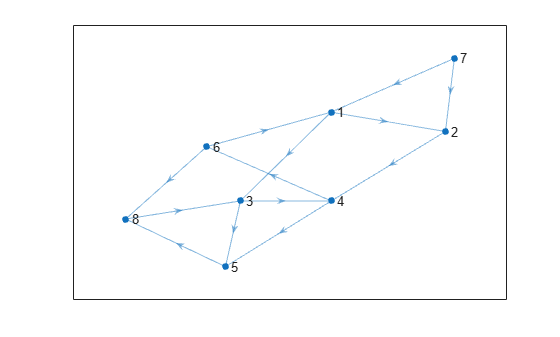Calculate the shortest path between nodes 7 and 8.

`P = shortestpath(G,7,8)`
```P = 1×5 7 1 3 5 8 ```

Create and plot a graph with weighted edges.

```s = [1 1 1 2 2 6 6 7 7 3 3 9 9 4 4 11 11 8]; t = [2 3 4 5 6 7 8 5 8 9 10 5 10 11 12 10 12 12]; weights = [10 10 10 10 10 1 1 1 1 1 1 1 1 1 1 1 1 1]; G = graph(s,t,weights); plot(G,'EdgeLabel',G.Edges.Weight)```Find the shortest path between nodes 3 and 8, and specify two outputs to also return the length of the path.

`[P,d] = shortestpath(G,3,8)`
```P = 1×5 3 9 5 7 8 ```
```d = 4 ```

Since the edges in the center of the graph have large weights, the shortest path between nodes 3 and 8 goes around the boundary of the graph where the edge weights are smallest. This path has a total length of 4.

Create and plot a graph with weighted edges, using custom node coordinates.

```s = [1 1 1 1 1 2 2 7 7 9 3 3 1 4 10 8 4 5 6 8]; t = [2 3 4 5 7 6 7 5 9 6 6 10 10 10 11 11 8 8 11 9]; weights = [1 1 1 1 3 3 2 4 1 6 2 8 8 9 3 2 10 12 15 16]; G = graph(s,t,weights); x = [0 0.5 -0.5 -0.5 0.5 0 1.5 0 2 -1.5 -2]; y = [0 0.5 0.5 -0.5 -0.5 2 0 -2 0 0 0]; p = plot(G,'XData',x,'YData',y,'EdgeLabel',G.Edges.Weight);```Find the shortest path between nodes 6 and 8 based on the graph edge weights. Highlight this path in green.

`[path1,d] = shortestpath(G,6,8)`
```path1 = 1×5 6 3 1 4 8 ```
```d = 14 ```
`highlight(p,path1,'EdgeColor','g')`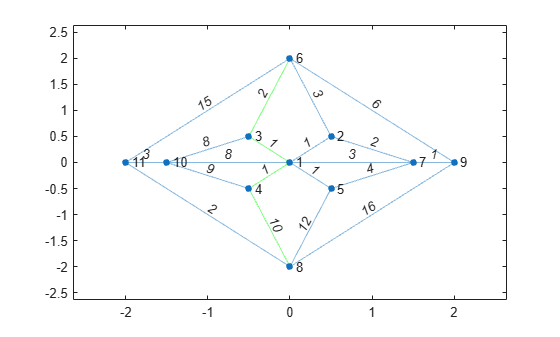Specify `Method` as `unweighted` to ignore the edge weights, instead treating all edges as if they had a weight of 1. This method produces a different path between the nodes, one that previously had too large of a path length to be the shortest path. Highlight this path in red.

`[path2,d] = shortestpath(G,6,8,'Method','unweighted')`
```path2 = 1×3 6 9 8 ```
```d = 2 ```
`highlight(p,path2,'EdgeColor','r')`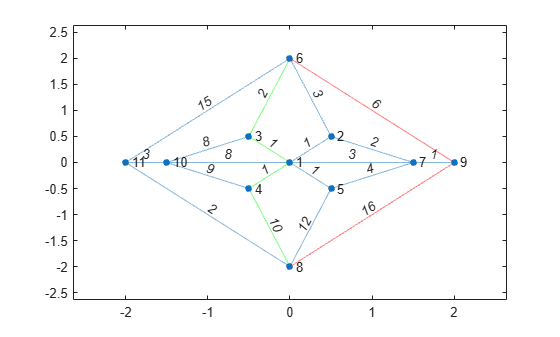Plot the shortest path between two nodes in a multigraph and highlight the specific edges that are traversed.

Create a weighted multigraph with five nodes. Several pairs of nodes have more than one edge between them. Plot the graph for reference.

```G = graph([1 1 1 1 1 2 2 3 3 3 4 4],[2 2 2 2 2 3 4 4 5 5 5 2],[2 4 6 8 10 5 3 1 5 6 8 9]); p = plot(G,'EdgeLabel',G.Edges.Weight);```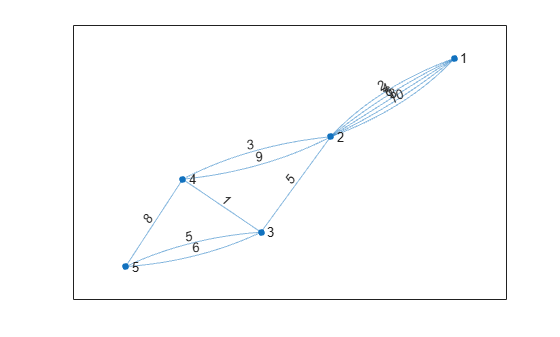Find the shortest path between node 1 and node 5. Since several of the node pairs have more than one edge between them, specify three outputs to `shortestpath` to return the specific edges that the shortest path traverses.

`[P,d,edgepath] = shortestpath(G,1,5)`
```P = 1×5 1 2 4 3 5 ```
```d = 11 ```
```edgepath = 1×4 1 7 9 10 ```

The results indicate that the shortest path has a total length of 11 and follows the edges given by `G.Edges(edgepath,:)`.

`G.Edges(edgepath,:)`
```ans=4×2 table EndNodes Weight ________ ______ 1 2 2 2 4 3 3 4 1 3 5 5 ```

Highlight this edge path by using the `highlight` function with the `'Edges'` name-value pair to specify the indices of the edges traversed.

`highlight(p,'Edges',edgepath)`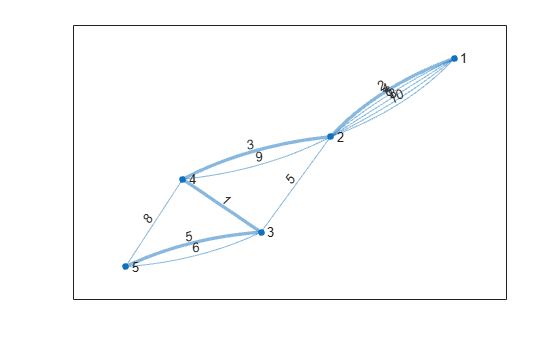## Input Arguments

collapse all

Input graph, specified as either a `graph` or `digraph` object. Use `graph` to create an undirected graph or `digraph` to create a directed graph.

Example: `G = graph(1,2)`

Example: `G = digraph([1 2],[2 3])`

Source and target node IDs, specified as separate arguments of node indices or node names.

ValueExample
Scalar node index`1`
Character vector node name`'A'`
String scalar node name`"A"`

Example: `shortestpath(G,2,5)` computes the shortest path between node 2 and node 5.

Example: `shortestpath(G,'node1','node2')` computes the shortest path between the named nodes `node1` and `node2`.

Shortest path algorithm, specified as one of the options in the table.

OptionDescription
`'auto'` (default)

The `'auto'` option automatically selects the algorithm:

• `'unweighted'` is used for `graph` and `digraph` inputs with no edge weights.

• `'positive'` is used for all `graph` inputs that have edge weights, and requires the weights to be nonnegative. This option is also used for `digraph` inputs with nonnegative edge weights.

• `'mixed'` is used for `digraph` inputs whose edge weights contain some negative values. The graph cannot have negative cycles.

`'unweighted'`

Breadth-First computation that treats all edge weights as `1`.

`'positive'`

Dijkstra algorithm that requires all edge weights to be nonnegative.

`'mixed'` (only for `digraph`)

Bellman-Ford algorithm for directed graphs that requires the graph to have no negative cycles.

While `'mixed'` is slower than `'positive'` for the same problem, `'mixed'` is more versatile as it allows some edge weights to be negative.

`'acyclic'` (only for `digraph`)

Algorithm designed to improve performance for directed, acyclic graphs (DAGs) with weighted edges.

Use `isdag` to confirm if a directed graph is acyclic.

Note

For most graphs, `'unweighted'` is the fastest algorithm, followed by `'acyclic'`, `'positive'`, and `'mixed'`.

Example: `shortestpath(G,s,t,'Method','acyclic')`

## Output Arguments

collapse all

Shortest path between nodes, returned as a vector of node indices or an array of node names. `P` is empty, `{}`, if there is no path between the nodes.

• If `s` and `t` contain numeric node indices, then `P` is a numeric vector of node indices.

• If `s` and `t` contain node names, then `P` is a cell array or string array containing node names.

If there are multiple shortest paths between `s` and `t`, then `P` contains only one of the paths. The path that is returned can change depending on which algorithm `Method` specifies.

Shortest path distance, returned as a numeric scalar. `d` is the summation of the edge weights between consecutive nodes in `P`. If there is no path between the nodes, then `d` is `Inf`.

Edges on shortest path, returned as a vector of edge indices. For multigraphs, this output indicates which edge between two nodes is on the path. This output is compatible with the `'Edges'` name-value pair of `highlight`, for example: `highlight(p,'Edges',edgepath)`.

## Tips

• The `shortestpath`, `shortestpathtree`, and `distances` functions do not support undirected graphs with negative edge weights, or more generally any graph containing a negative cycle, for these reasons:

• A negative cycle is a path that leads from a node back to itself, with the sum of the edge weights on the path being negative. If a negative cycle is on a path between two nodes, then no shortest path exists between the nodes, since a shorter path can always be found by traversing the negative cycle.

• A single negative edge weight in an undirected graph creates a negative cycle.